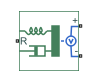# Accelerometer

Behavioral model of MEMS accelerometer

•Libraries:
Simscape / Electrical / Sensors & Transducers

## Description

The Accelerometer block implements a behavioral model of a MicroElectroMechanical Systems (MEMS) accelerometer. For the default output type `Voltage level`, the accelerometer provides an output voltage that is proportional to the acceleration rate presented at the mechanical translational physical port R. The output voltage is limited according to the values that you provide for maximum and minimum output voltage.

The block also has an alternative output type, ```PWM duty cycle```. With this choice, the output of the block is a PWM signal with a duty cycle that is proportional to the measured acceleration. You can limit the variation in duty cycle to a specified range.

Optionally, you can model sensor dynamics by setting the Dynamics parameter to `Model sensor bandwidth`. Including dynamics adds a first-order lag between the angular rate presented at port R and the corresponding voltage applied to the electrical + and - ports.

If running your simulation with a fixed-step solver, or generating code for hardware-in-the-loop testing, MathWorks recommends that you set the Dynamics parameter to ```No dynamics — Suitable for HIL```, because this avoids the need for a small simulation time step if the sensor bandwidth is high.

### Variables

To set the priority and initial target values for the block variables prior to simulation, use the Initial Targets section in the block dialog box or Property Inspector. For more information, see Set Priority and Initial Target for Block Variables.

Nominal values provide a way to specify the expected magnitude of a variable in a model. Using system scaling based on nominal values increases the simulation robustness. Nominal values can come from different sources, one of which is the Nominal Values section in the block dialog box or Property Inspector. For more information, see System Scaling by Nominal Values.

The Measured acceleration variable target specifies the initial output for the sensor.

## Ports

### Conserving

expand all

Mechanical conserving port associated with the accelerometer translational port.

Electrical conserving port associated with the accelerometer positive terminal.

Electrical conserving port associated with the accelerometer negative terminal.

## Parameters

expand all

Select one of the following options to define the block output type:

• `Voltage level` — The amplitude of the output voltage is proportional to the measured acceleration. This is the default option.

• `PWM duty cycle` — The duty cycle (on time divided by the pulse total time) is proportional to the measured acceleration.

Change in output voltage level per unit change in acceleration when the output is not being limited.

#### Dependencies

This parameter is visible only when you select ```Voltage level``` for the Output type parameter.

The output voltage from the sensor when the acceleration is zero.

#### Dependencies

This parameter is visible only when you select ```Voltage level``` for the Output type parameter.

The maximum output voltage from the sensor, which determines the sensor maximum measured positive acceleration.

#### Dependencies

This parameter is visible only when you select ```Voltage level``` for the Output type parameter.

The minimum output voltage from the sensor, which determines the sensor maximum measured negative acceleration. This parameter is only visible when you select `Voltage level` for the Output type parameter. The default value is `1` V.

The change in duty cycle per unit acceleration. Duty cycle is expressed as a percentage of the PWM period.

#### Dependencies

This parameter is visible only when you select ```PWM duty cycle``` for the Output type parameter.

The duty cycle output by the sensor when the acceleration is zero.

#### Dependencies

This parameter is visible only when you select ```PWM duty cycle``` for the Output type parameter.

The maximum duty cycle output by the sensor. Increasing acceleration levels beyond this point will not register an increase in duty cycle.

#### Dependencies

This parameter is visible only when you select ```PWM duty cycle``` for the Output type parameter.

The minimum duty cycle output by the sensor. Decreasing acceleration levels beyond this point will not register a decrease in duty cycle.

#### Dependencies

This parameter is visible only when you select ```PWM duty cycle``` for the Output type parameter.

The frequency of the output pulse train.

#### Dependencies

This parameter is visible only when you select ```PWM duty cycle``` for the Output type parameter.

The amplitude of the output pulse train when high.

#### Dependencies

This parameter is visible only when you select ```PWM duty cycle``` for the Output type parameter.

Select one of the following options for modeling sensor dynamics:

• ```No dynamics — Suitable for HIL``` — Do not model sensor dynamics. Use this option when running your simulation fixed step or generating code for hardware-in-the-loop testing, because this avoids the need for a small simulation time step if the sensor bandwidth is high. This is the default option.

• `Model sensor bandwidth` — Model sensor dynamics with a first-order lag approximation, based on the Bandwidth parameter value. You can control the initial condition for the lag by specifying the Measured acceleration variable target.

Specifies the 3dB bandwidth for the measured acceleration assuming a first-order time constant.

#### Dependencies

This parameter is visible only when you select ```Model sensor bandwidth``` for the Dynamics parameter.

## Version History

Introduced in R2012b